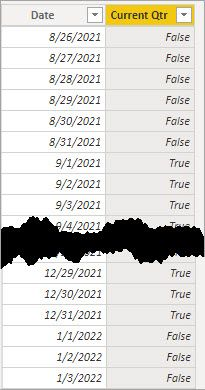cancel
Showing results for
Did you mean:## Dynamic Current Quarter Filter

Hi all,

I have a scenario where I want to apply a dynamic page filter to include only sales data in the current quarter. For example, today being 14 October 2021, I only want to see sales in this quarter (October 2021 - December 2021). Throughout November and December the filter would remain the same (October 2021 - December 2021) and would change on the 1st of January 2022 where it would change to filter sales only for January 2022 - March 2022 and so on.

Model is fairly straight forward (Sales fact table and sales date dimension table). I was thinking of possibly adding a custom true/false column on the sales date dimension table to indicate whether a given date was in the current quarter but am a bit stuck on how.

Looking for any suggestions on how to approach this. Can provide more info if needed.

Thank you

1 ACCEPTED SOLUTIONSuper User

My apologies, I needed to shift the moths a bit.  Give this a try:

``````Current Qtr =
VAR _StartOfQtr = DATE ( YEAR ( TODAY() ), FLOOR ( MONTH ( TODAY() ) -1 , 3 ) + 1 , 1 )
VAR _EndOfQtr = EOMONTH ( _StartOfQtr, 2 )
RETURN
[Date] >= _StartOfQtr && [Date] <= _EndOfQtr ``````
15 REPLIES 15Super User

We should just need to extend the number of months figuring into the end date like this.

``````Current Qtr =
VAR _StartOfQtr = DATE ( YEAR ( TODAY() ), FLOOR ( MONTH ( TODAY() ) -1 , 3 ) + 1 , 1 )
VAR _End = EOMONTH ( _StartOfQtr, 11 )
RETURN
[Date] >= _StartOfQtr && [Date] <= _End``````Super User

Simple enough, add a column of Year+Qtr in the date table this way,

``YrQtr = Year( DATES[Date] ) * 10 + Quarter( Dates[Date] )``

 Thanks to the great efforts by MS engineers to simplify syntax of DAX! Most beginners are SUCCESSFULLY MISLED to think that they could easily master DAX; but it turns out that the intricacy of the most frequently used RANKX() is still way beyond their comprehension! DAX is simple, but NOT EASY!Super User

``````Current Qtr =
VAR _StartOfQtr = DATE ( YEAR ( TODAY() ), FLOOR ( MONTH ( TODAY() ) -1 , 3 ), 1 )
VAR _EndOfQtr = EOMONTH ( _StartOfQtr, 3 )
RETURN
[Date] >= _StartOfQtr && [Date] <= _EndOfQtr``````Then just set a filter on that column for True.  When the date rolls into next quarter the dates flagged True will shift to the new quarter.Hi,

Thank you. This is close however, _StartOfQtr is returning the 1st of September as the start of the quarter resulting in four months instead of the quarter starting on the 1st of October.Super User

My apologies, I needed to shift the moths a bit.  Give this a try:

``````Current Qtr =
VAR _StartOfQtr = DATE ( YEAR ( TODAY() ), FLOOR ( MONTH ( TODAY() ) -1 , 3 ) + 1 , 1 )
VAR _EndOfQtr = EOMONTH ( _StartOfQtr, 2 )
RETURN
[Date] >= _StartOfQtr && [Date] <= _EndOfQtr ``````Regular Visitor

Hello - May I ask for a formula that would return "the current and next 3 Quarters", please?

Thank you!Frequent Visitor

Hey, is there a way to reuse this for last two quarters? Meaning current + last quarter?Super User

That would look somehting like this.

``````Current Qtr =
VAR _StartOfQtr = DATE ( YEAR ( TODAY() ), FLOOR ( MONTH ( TODAY() ) -1 , 3 ) + 1 , 1 )
VAR _StartOfPQtr = EOMONTH ( _StartOfQtr, -4 ) +1
VAR _EndOfQtr = EOMONTH ( _StartOfQtr, 2 )
RETURN
[Date] >= _StartOfPQtr && [Date] <= _EndOfQtr``````Anonymous
Not applicable

Hi ,
Even I have the same requirement. But as per my requirement ,I need to calculate " current quarter + Next quarter " sale.Super User

@Anonymous

For that we would just need this.

``````Current Qtr =
VAR _StartOfQtr = DATE ( YEAR ( TODAY() ), FLOOR ( MONTH ( TODAY() ) -1 , 3 ) + 1 , 1 )
VAR _EndOfNextQtr = EOMONTH ( _StartOfQtr, 5 )
RETURN
[Date] >= _StartOfQtr && [Date] <= _EndOfNextQtr``````Frequent Visitor

Hi, can I have the formula but for just previous quarter only please? I would really appreciate it.Super User

That would be something like this.

``````Last Qtr =
VAR _StartOfQtr = DATE ( YEAR ( TODAY() ), FLOOR ( MONTH ( TODAY() ) -1 , 3 ) + 1 , 1 )
VAR _StartOfLastQtr = EOMONTH ( _StartOfQtr, -4 ) + 1
VAR _EndOfLastQtr = EOMONTH ( _StartOfLastQtr, 2 )
RETURN
[Date] >= _StartOfLastQtr && [Date] <= _EndOfLastQtr``````Frequent Visitor

Thank you!Perfect thank you!Super User

@MBPCCX , This Qtr vs last qtr based on today

This Qtr =
var _max = maxx(allselected('Date'), 'Date'[Date])
var _month = mod(month(_max),3)
var _min = date(year(_max),month(_max) -1* if(_month=0,3,_month) ,1)
var _day = date(year(_min),month(_min)+3 ,1) -1
return
CALCULATE(sum('Table'[Qty]), FILTER(ALL('Date'),'Date'[Date] >=_min && 'Date'[Date] <= _day) )

Last Qtr =
var _max = maxx(allselected('Date'), 'Date'[Date])
var _month = mod(month(_max),3)
var _min = date(year(_max)-1,month(_max) -1* if(_month=0,3,_month) ,1)
var _day = date(year(_min),month(_min)+3 ,1) -1
return
CALCULATE(sum('Table'[Qty]), FILTER(ALL('Date'),'Date'[Date] >=_min && 'Date'[Date] <= _day) )

based on selected date

Qtr Sales = CALCULATE(SUM(Sales[Sales Amount]),DATESQTD(ENDOFQUARTER('Date'[Date])))

Last QUARTER Sales = CALCULATE(SUM(Sales[Sales Amount]),DATESQTD( ENDOFQUARTER(dateadd('Date'[Date],-1,QUARTER))))
Last QUARTER Sales = CALCULATE(SUM(Sales[Sales Amount]),PREVIOUSQUARTER(('Date'[Date])))

To get the best of the time intelligence function. Make sure you have a date calendar and it has been marked as the date in model view. Also, join it with the date column of your fact/s. Refer :radacad sqlbi My Video Series Appreciate your Kudos.